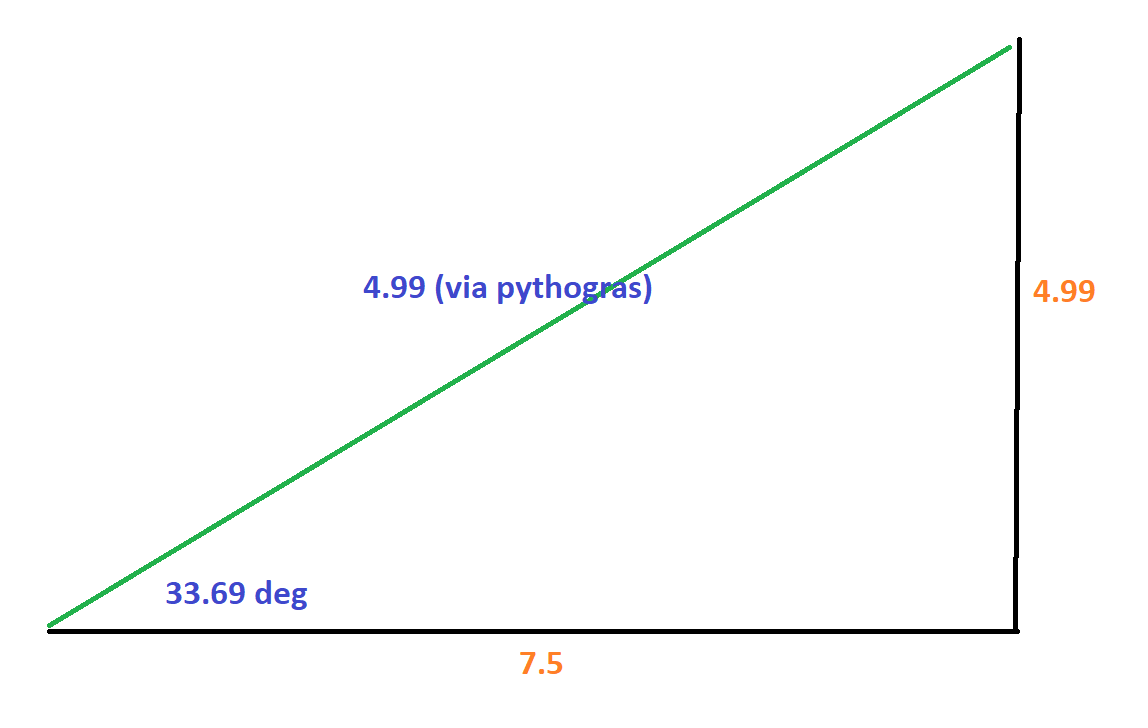# Thread: Vector addition

1. ## Vector additionHad this problem with vector addition don’t judgeQuestion: find the sum of vector a and b
Components of vA
Sin30=x/15
X=15*sin30=7.5
Cos30=-y/15
y=-12.9

Components of vB
Tan45=x/8
X=8

THIS IS THE PART I STALLED DUNNO HOW TO FIND Y COMPONENT OF vB below I have attached a diagram of how I did the question.ThanksReply With Quote

2. ## Re: Vector addition

just to confirm your question is find (vector A + vector B)?

it is good to see that you have had a go at the question first

so let's break up the components of the vectors.

for vector A, we can see that the it points straight down on the y axis. There is no X component of this vector.

for vector B, we can use trig to resolve the vectors. the length of the Y component of Vb will be 15cos(30) = 12.99m and the X component of Vb will be 15sin(30) = 7.5m

Your Y component for vector B has a negative for some reason. It would be best to take one direction of each axis as positive. So conventionally up on the y axis and to the right on the X axis are positive.

Now what we do is add the X components of Vector A and Vector B together AND then the Y components of Vector A and Vector B together to get the resultant X and Y vector components.

for X direction: 7.5m + 0m = 7.5m (note that there was no X component for vector B)

for Y direction: -8m + 12.99m = 4.99m (-8m because the vector is pointing down)

So the resultant vector will look like this (in green):so the final answer would be:

the resultant vector is of length 4.99 units at 33.69 degrees CCW from the positive X axisReply With Quote

#### Thread Information

##### Users Browsing this Thread

There are currently 1 users browsing this thread. (0 members and 1 guests)

####Posting Permissions

• You may not post new threads
• You may not post replies
• You may not post attachments
• You may not edit your posts
•